# RunKit +#### Try any Node.js package right in your browser

This is a `playground` to test code. It runs a full `Node.js` environment and already has all of `npm`’s 1,000,000+ packages pre-installed, including `ola` with all `npm` packages installed. Try it out:

var ola = require("ola")

This service is provided by RunKit and is not affiliated with npm, Inc or the package authors.

# OlaSmooth animation library for inbetweening / interpolating numbers in realtime: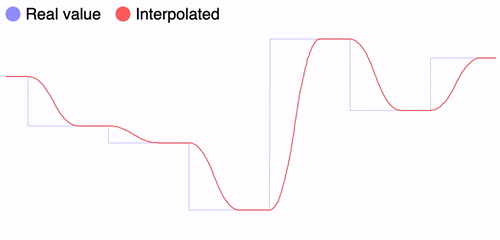``````// Start tracking the value
const pos = Ola({ y: 0 });

// Set the value to update async
pos.set({ y: 100 });

// Read the evolution over time
setInterval(() => graph(pos.y), 5);
``````

It works with multiple values/dimensions: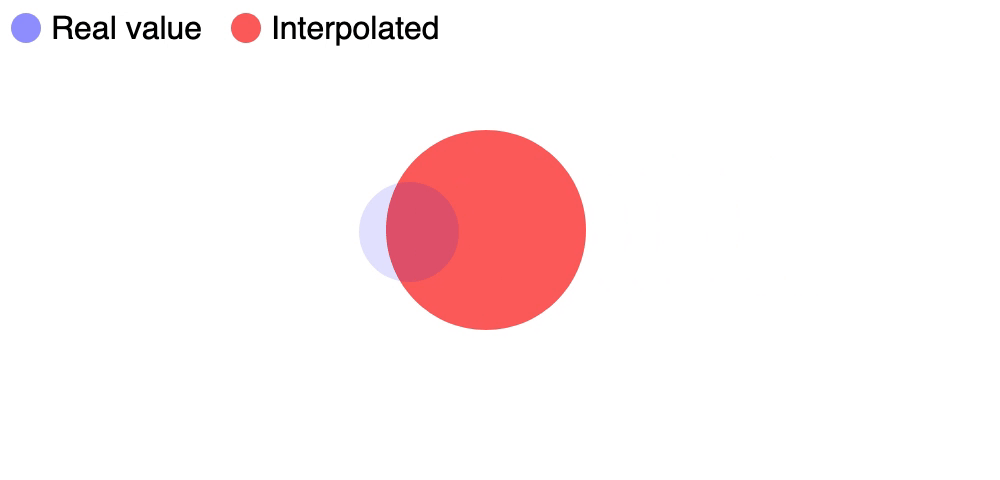``````const pos = Ola({ x: 0, y: 0 });

pos.set({ x: e.pageX, y: e.pageY });
});

setInterval(() => {
ball.style.left = `\${pos.x}px`;
ball.style.top = `\${pos.y}px`;
}, 10);
``````

Also works great with many instances since they are independent: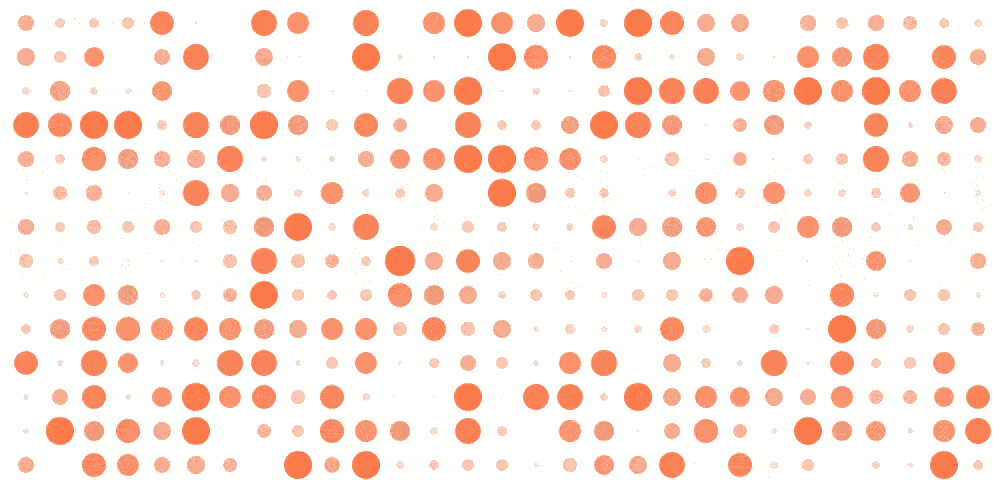``````// Generates 1000 instances seamlessly
const dots = Ola(Array(1000).fill(0));

setInterval(() => dots.forEach((dot, i) => {
dots[i] = Math.random();
}), 600);

// ... read + paint screen here
``````

Tip: click on the GIFs for a live demo with the code :)

## Getting started

Install it with npm:

``````npm install ola
``````

Then import it and use it:

``````import Ola from "ola";
const pos = Ola({ x: 0 });
console.log(pos.x); // 0
``````

If you prefer to use a CDN:

``````<script src="https://cdn.jsdelivr.net/npm/ola"></script>
<script type="text/javascript">
const pos = Ola({ x: 0 });
console.log(pos.x); // 0
</script>
``````

## Documentation

There are three distinct operations that can be run: creating an instance, setting it to update and reading it.

### Create an instance

``````Ola(initial, time = 300);
``````

The first parameter is the initial value. It can be either a single number, or an object of `key:numbers` or an array of numbers:

``````const heater = Ola(20); // Alias of `{ value: 20 }`
const motor = Ola({ angle: 180 }); // A named parameter for clarity
const position = Ola({ x: 0, y: 0 }); // Any number of properties
const heights = Ola([0, 0, 0, 0]); // A group of heights
``````

The second parameter is how long the transition will last. It should be a number that represents the time in milliseconds:

``````const heater = Ola(20); // Default = 300 ms
const motor = Ola({ angle: 180 }, 1000); // Turn the motor slowly
const position = Ola({ x: 0, y: 0 }, 100); // Quick movements for the position
const heights = Ola([0, 0, 0, 0], 300); // 300, same as the default
``````

Passing a single number as a parameter is the same as passing `{ value: num }`, we are just helping by setting a shortname. It is offered for convenience, but recommend not mixing both styles in the same project.

It works with Javascript numbers, but please keep things reasonable (under `Number.MAX_VALUE / 10`):

``````console.log(Ola(100));
console.log(Ola(-100));
console.log(Ola(0.001));
console.log(Ola(1 / 100));
``````

The time it takes to update can also be updated while setting the value, which will update it for any subsequent transition:

``````// All `pos.set()` will take 1 full second
const pos = Ola({ x: 0 }, 1000);
pos.set({ x: 100 }, 3000);
``````

### Update the value

``````heater.value = 25; // Since the constructor used a number, use `.value`
motor.angle = 90; // Turn -90 degrees from before
position.set({ x: 100, y: 100 }); // Move 0,0 => 100,100
heights = 120; // Move the second (0-index) item to 120
``````

When we update a property it is not updated instantaneously (that's the whole point of this library), but instead it's set to update asynchronously:

``````const pos = Ola({ x: 0 });
pos.set({ x: 100 });

// 0 - still hasn't updated
console.log(pos.x);

// 100 - after 300ms it's fully updated
setTimeout(() => console.log(pos.x), 1000);
``````

Remember that if you set the value as `Ola(10)`, this is really an alias for `Ola({ value: 10 })`, so use the property `.value` to update it:

``````heater.value = 25;
heater.set({ value: 25 });
``````

You can see in this graph, the blue line is the value that is set though `.set()`, while the red line is the value that reading it returns:``````log(heater.value); // Since the constructor used a number, use `.value`
log(motor.angle); // Read as an object property
log(position.get("x")); // Find the X value
log(heights); // Move the first item to 120
``````

You can read the value at any time, and the value will be calculated at that moment in time:

``````const pos = Ola({ x: 0 });
pos.set({ x: 100 });

setInterval(() => {
// It will update every time it's read
console.log(pos.x);
}, 10);
``````

In contrast to other libraries, there's no need to tick/update the function every N ms or before reading the value, since `Ola()` uses math functions you should just read it when needed.

## Features

While there are some other great libraries like Tween, this one has some improvements:

### Smooth in realtime

Other libraries don't move smoothly when there's an update while the previous transition is still ongoing. Ola makes sure there are no harsh corners: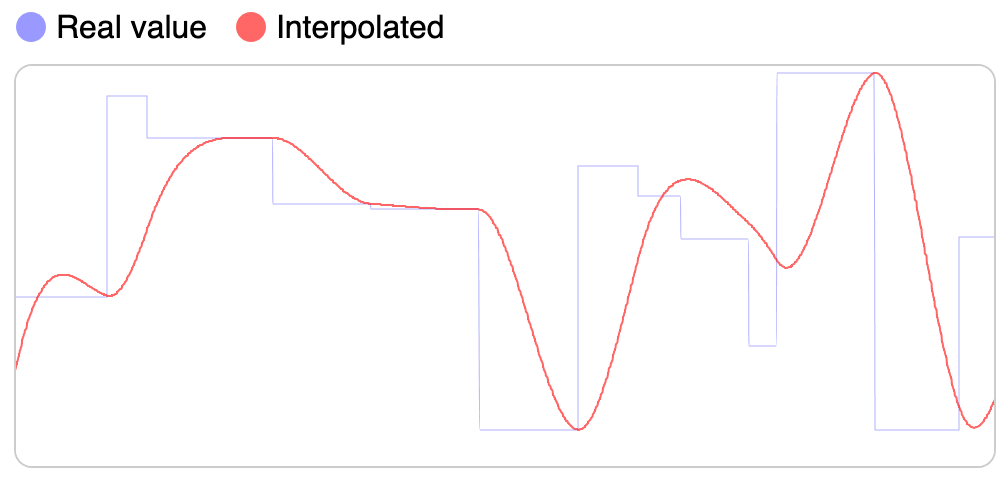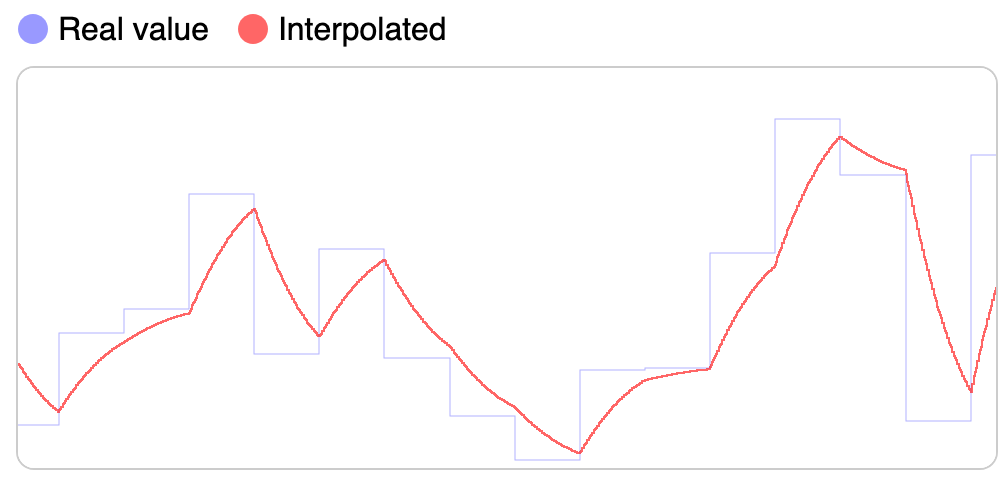Smooth interpolation with Ola() Harsh interpolation with Tweenmax

Status of libraries updating animation mid-way:

• Ola.js - working smoothly, see screenshot above.
• TweenMax - harsh transition. See screenshot above.
• Tween.js - no transitions at all, feature request made in 2016: https://github.com/tweenjs/tween.js/issues/257
• Open an Issue with other libraries that you know.

Since this is driven by mathematical equations, the library doesn't calculate any value until it needs to be read/updated. It will also only change the one we need instead of all of the values:

``````const position = Ola({ x: 0, y: 0 });
position.x = 10; // Only updates X
console.log(position.x); // Calculates only X position, not y
``````

Not only this is great for performance, but it also makes for a clean self-contained API where each instance is independent and portable.

## Others from Author

Like this project? Francisco has many more! Check them out:

• server.js - a batteries-included Node.js server
• translate.js - to easily translate text on the browser and Node.js